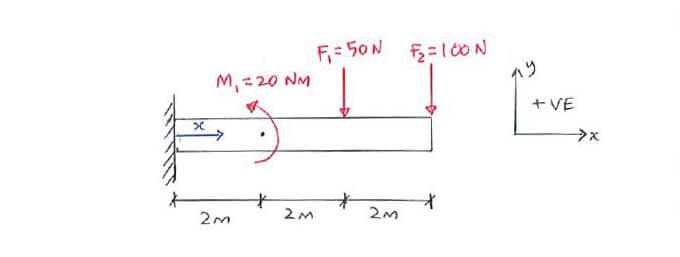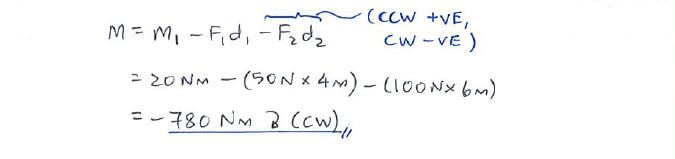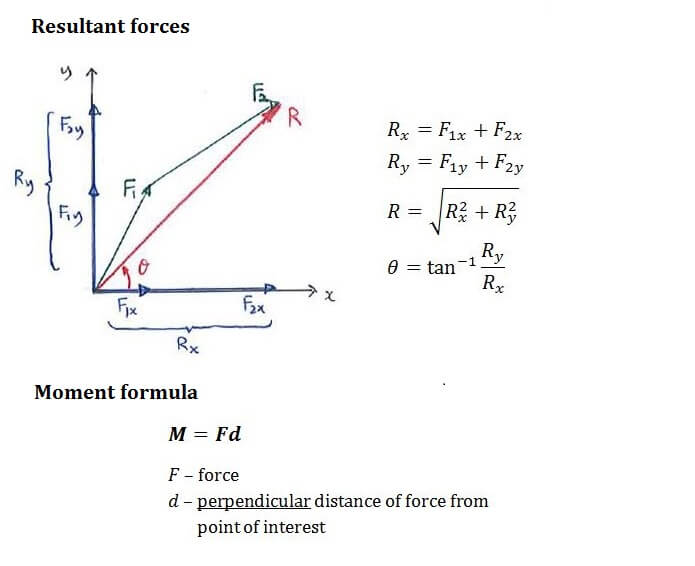# Example QuestionClick below to show answer. X

#### Step 1: Calculate resultant force Step 1: Calculate resultant force#### Step 2: Calculate net moment Step 2: Calculate net moment#### Useful formula: Useful formula:Now that you've mastered this question, you can try Question 1.